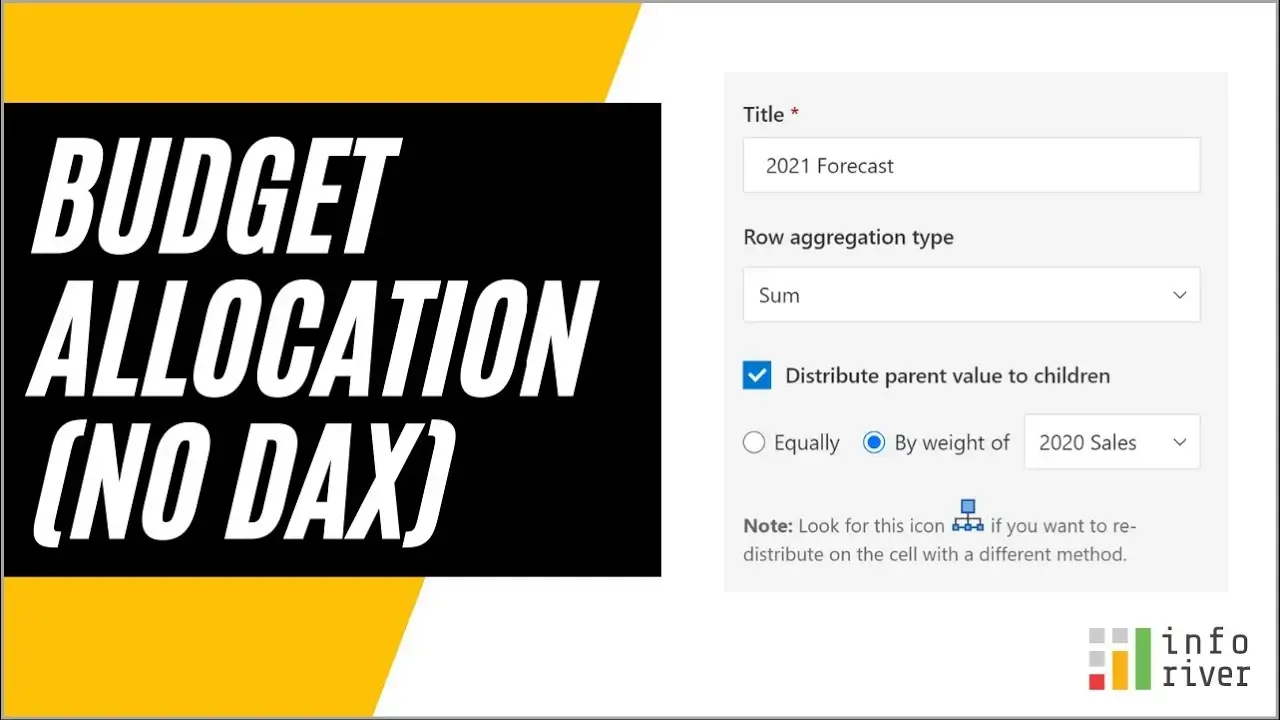Upcoming webinar on 'Inforiver Charts : The fastest way to deliver stories in Power BI', Aug 29th , Monday, 10.30 AM CST.    Register Now

# Calculate remaining budget in Power BI (without using DAX)

by Chandra Vadana Rajamohan | Nov 15, 2021 |

In Microsoft Power BI, you may often encounter incorrect total values while using calculated measures. Here is one such example. We have budget amounts for a few products (Budget Amount) and have incurred expenses against each (Posted Expenses). Our objective is to calculate remaining budget in a new column, and add them up.

Let us calculate Remaining Budget using the formula = Posted Expenses – Budget Amount. We need to do this only for rows where expenses don’t exceed the budget (else we will have negative remaining budgets). The correct logical expression is IF (Budget < Expenses, “”, Budget-Expenses)

The DAX equivalent is

Budget Remaining = IF (SUM ([Budget Amount])

However, notice that the total budget is incorrect. Instead of showing 4000 (sum of individual records), it shows 2500 (the difference between Budget Amount Totals and Posted Expenses Totals).

The traditional fix for this using DAX has been as follows.

## Calculate remaining budget using DAX

There are several ways to do this – using COUNTROWS or HASONEFILTER:

`Budget Remaining Correct = IF (COUNTROWS (VALUES ([Product ID])) =1, [Budget Remaining], SUMX (VALUES ([Product ID]), [Budget Remaining])`
`Budget Remaining Correct = IF (HASONEFILTER ([Product ID]), [Budget Remaining], SUMX (VALUES ([Product ID]), [Budget Remaining]))`

There are other solutions such as using virtual tables based on the complexity of your requirements. But there is one other solution – which is most straightforward – using Inforiver.

## Calculate remaining budget without using DAX – The Inforiver Way

In Inforiver, we create a formula measure using a simple IF statement.

There is just one more setting that you need to turn on – the Row Aggregation Type. By default, it is set to Formula – meaning, it applies the same formula on totals & subtotals. But we want to avoid just that.

Now change the Row Aggregation Type to type Sum – This ensures that the individual rows are simply added up to calculate totals. There are few other row aggregation types as well that you can try out, depending on your need.

And we get the values right, and this method is a lot faster than using DAX.

Watch this video how to efficiently allocate budgets without using DAXStart a free trial of Inforiver today!